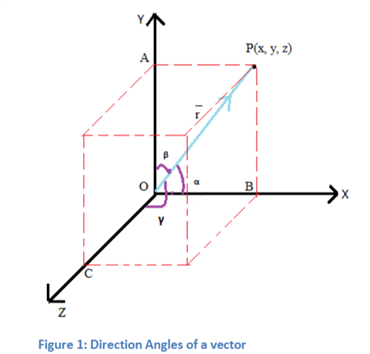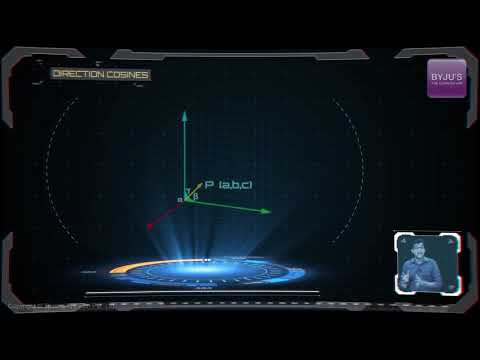## Direction Cosines

When a directed line OP passing through the origin makes

$$\begin{array}{l}\alpha \end{array}$$
,
$$\begin{array}{l}\beta\end{array}$$
and
$$\begin{array}{l} \gamma\end{array}$$
angles with the
$$\begin{array}{l}x\end{array}$$
,
$$\begin{array}{l}y \end{array}$$
and
$$\begin{array}{l}z \end{array}$$
axis respectively with O as the reference, these angles are referred as the direction angles of the line and the cosine of these angles give us the direction cosines. These direction cosines are usually represented as l, m and n.If we extend the line  OP on the three dimensional Cartesian coordinate system, then to figure out the direction cosines, we need to take the supplement of the direction angles. It is pretty obvious from this statement that on reversal of the  line  OP in opposite direction, the direction cosines of the line also get reversed. In a situation where the given line does not pass through the origin, a line parallel to the given line passing through the origin is drawn and in doing so, the angles remain same as the angles made by the original line. Hence, we get the same direction.

Since we are considering a line passing through the origin to figure out the direction angles and their cosines, we can consider the position vectors of the line OP.

If  OP = r, then from the above figure 1, we can see that

$$\begin{array}{l}x \end{array}$$
=
$$\begin{array}{l}r cos \alpha\end{array}$$

$$\begin{array}{l}y\end{array}$$
=
$$\begin{array}{l} r cos \beta\end{array}$$

$$\begin{array}{l}z\end{array}$$
=
$$\begin{array}{l} r cos \gamma\end{array}$$

Where r  denotes the magnitude of the vector and it is given by,

$$\begin{array}{l}r\end{array}$$
=
$$\begin{array}{l}\sqrt{(x – 0)^2 + (y – 0)^2 + (z – 0)^2}\end{array}$$

$$\begin{array}{l}\Rightarrow r\end{array}$$
=
$$\begin{array}{l} \sqrt{x^2 + y^2 + z^2}\end{array}$$

The cosines of direction angles are given by

$$\begin{array}{l}cos \alpha\end{array}$$
,
$$\begin{array}{l}cos \beta\end{array}$$
and
$$\begin{array}{l}cos\gamma\end{array}$$
and these are denoted by l, m and n respectively. Therefore, the above equations can be reframed as:

$$\begin{array}{l}x\end{array}$$
=
$$\begin{array}{l}r cos\alpha\end{array}$$
=
$$\begin{array}{l} lr\end{array}$$
——————————————————— (1)

$$\begin{array}{l}y\end{array}$$
=
$$\begin{array}{l} r cos\beta\end{array}$$
=
$$\begin{array}{l} mr \end{array}$$
——————————————————– (2)

$$\begin{array}{l}z\end{array}$$
=
$$\begin{array}{l}r cos \gamma\end{array}$$
=
$$\begin{array}{l} nr \end{array}$$
——————————————————— (3)

We can also represent  r in terms of its unit vector components using the orthogonal system.

$$\begin{array}{l}r\end{array}$$
=
$$\begin{array}{l} x\hat{i} + y \hat{j} + k \hat{z}\end{array}$$

Substituting the values of x, y and z, we have

$$\begin{array}{l}r \end{array}$$
=
$$\begin{array}{l} lr\hat{i} + mr\hat{j} + nr\hat{z}\end{array}$$

$$\begin{array}{l}\Rightarrow \hat{r}\end{array}$$
=
$$\begin{array}{l} \frac{r}{|r|}\end{array}$$
=
$$\begin{array}{l} l\hat{i} + m\hat{j} + n \hat{z}\end{array}$$

Therefore, we can say that cosines of direction angles of a vector are the coefficients of the unit vectors

$$\begin{array}{l}\hat{i}\end{array}$$
,
$$\begin{array}{l} \hat{j}\end{array}$$
and
$$\begin{array}{l}\hat{k}\end{array}$$
when the unit vector
$$\begin{array}{l}\hat{r}\end{array}$$
is resolved in terms of its rectangular components.

Any number proportional to the direction cosine is known as the direction ratio of a line. These direction numbers are represented by a, b and c.

Also as

$$\begin{array}{l}OP^2\end{array}$$
=
$$\begin{array}{l} OA^2 + OB^2 + OC^2 \end{array}$$

In simple terms,

$$\begin{array}{l}r\end{array}$$
=
$$\begin{array}{l}\sqrt{x^2 + y^2 + z^2}\end{array}$$

On dividing the equation,

$$\begin{array}{l}r^2 \end{array}$$
=
$$\begin{array}{l} x^2 + y^2 + z^2~ by ~r^2 \end{array}$$
we have,

$$\begin{array}{l}\frac{r^2}{r^2} \end{array}$$
=
$$\begin{array}{l} \frac{x^2}{r^2} + \frac{y^2}{r^2} + \frac{z^2}{r^2}\end{array}$$

Using equations 1, 2 and 3, we get

$$\begin{array}{l}\Rightarrow 1 \end{array}$$
=
$$\begin{array}{l}(\frac{x}{r})^2 + (\frac{y}{r})^2 + (\frac{z}{r})^2\end{array}$$
=
$$\begin{array}{l}l^2 + m^2 + n^2\end{array}$$

We can conclude that sum of the squares of the direction cosines of a line is 1.

From the above definition, we can say that

$$\begin{array}{l}a ∝ l\end{array}$$

$$\begin{array}{l}b ∝ m\end{array}$$

$$\begin{array}{l}c ∝ n\end{array}$$

From these relations, we get

$$\begin{array}{l} a \end{array}$$
=
$$\begin{array}{l} kl\end{array}$$

$$\begin{array}{l} b \end{array}$$
=
$$\begin{array}{l} km\end{array}$$

$$\begin{array}{l} c \end{array}$$
=
$$\begin{array}{l} kn\end{array}$$

The ratio between the direction cosines and direction ratios of a line is given by

$$\begin{array}{l}\frac{l}{a} \end{array}$$
=
$$\begin{array}{l}\frac{m}{b}\end{array}$$
=
$$\begin{array}{l}\frac{n}{c}\end{array}$$
=
$$\begin{array}{l}k\end{array}$$

But we know that

$$\begin{array}{l}l^2 + m^2 + n^2 \end{array}$$
=
$$\begin{array}{l}1\end{array}$$

From this, we can find that

$$\begin{array}{l}k\end{array}$$
=
$$\begin{array}{l} \pm \frac{1}{\sqrt{a^2 + b^2 + c^2}}\end{array}$$

The value of k can be chosen as positive or negative depending upon the direction of the directed line.

We can take any number of direction ratios by altering the value of k.
Also check: How to check Direction Cosine and Direction ratioWe are clear on the concept of the direction ratios and cosines of a line. To investigate more about three-dimensional geometry, download BYJU’S learning app and keep learning.